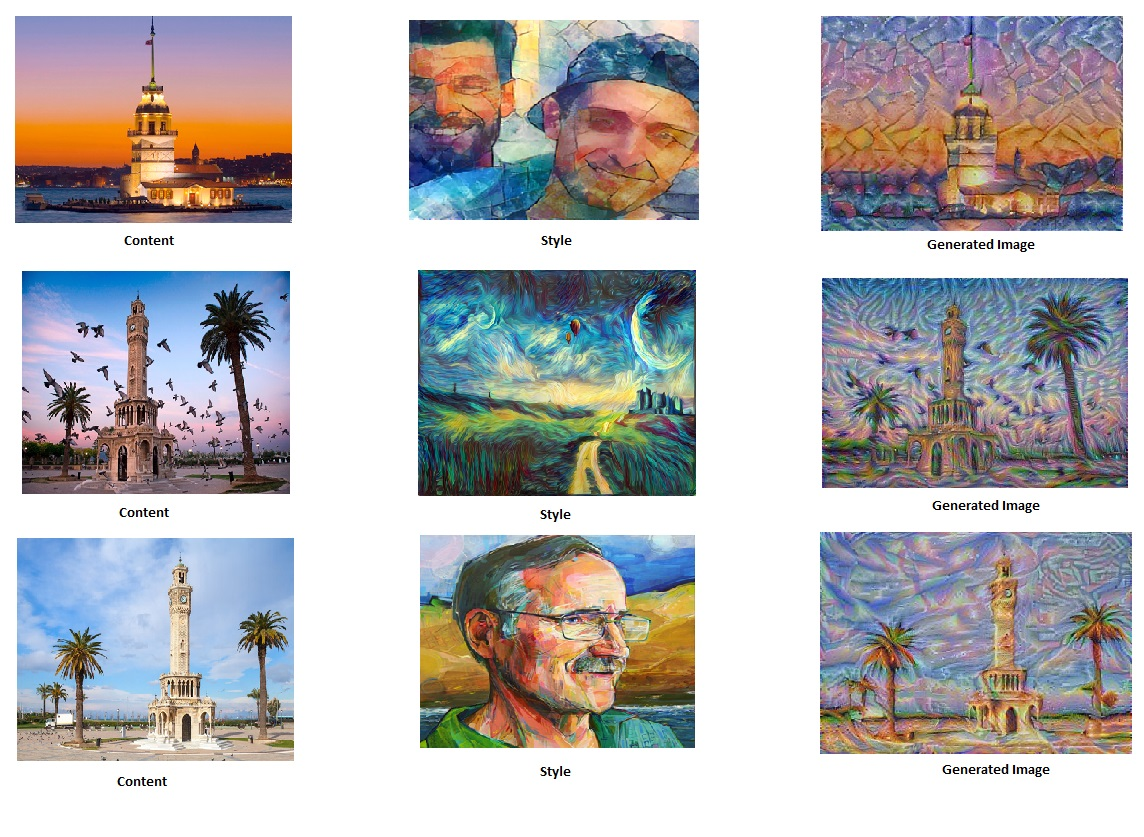# Neural Style Transfer Implementation

Neural Style Transfer is a method of creating artistic style images using Deep Neural Networks (Convolutional Neural Networks). This algorithm was created by Gatys et al. (2015) (https://arxiv.org/abs/1508.06576). Code is adapted from Andrew Ng’s Course ‘Convolutional Neural Networks”.

## Results## Transfer Learning

“Following the original NST paper (https://arxiv.org/abs/1508.06576), we will use the VGG network. Specifically, we’ll use VGG-19, a 19-layer version of the VGG network. This model has already been trained on the very large ImageNet database, and thus has learned to recognize a variety of low level features (at the earlier layers) and high level features (at the deeper layers)”

## Neural Style Transfer Algorithm

• Calculate the content cost function
• Calculate the style cost function
• Put it together to get $J(G) = \alpha J_{content}(C,G) + \beta J_{style}(S,G)$.
• Create an Interactive Session (tensorflow)
• Randomly initialize the image to be generated
• Build the TensorFlow graph:
• Run the content image through the VGG16 model and compute the content cost
• Run the style image through the VGG16 model and compute the style cost
• Compute the total cost
• Define the optimizer and the learning rate
• Initialize the TensorFlow graph and run it for a large number of iterations, updating the generated image at every step.

## References